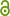# Enhanced FDTD edge correction for nonlinear effects calculation

C. Classen, J. Förstner, T. Meier, R. Schuhmann, in: 2010 IEEE Antennas and Propagation Society International Symposium, IEEE, 2010.2010 Classen,Förstner, Meier T,Schuhmann_Enhanced FDTD edge correction for nonlinear effects calculation.pdf 209.41 KB
URN
Conference Paper | Published | English
Author
Abstract
The electromagnetic field in the vicinity of sharp edges needs a special treatment in numeric calculation whenever accurate, fast converging results are necessary. One of the fundamental works concerning field singularities has been proposed in 1972  and states that the electromagnetic energy density must be integrable over any finite domain, even if this domain contains singularities. It is shown, that the magnetic field H(, ϕ) and electric field E(, ϕ) are proportional to ∝ (t−1) for  → 0. The variable  is the distance to the edge and t has to fulfill the integrability condition and thus is restricted to 0 < t < 1. This result is often used to reduce the error corresponding to the singularity without increasing the numerical effort [2 - 5]. For this purpose, a correction factor K is estimated by inserting the proportionality into the wave equation. It is shown, that this method improves the accuracy of the result significantly, however the order of convergence is often not studied. In  a method to modify the material parameters in order to use analytic results to improve the numeric calculation is presented. In this contribution we will - inspired by the scheme given in  - develop a new method to estimate a correction factor for perfect conducting materials (PEC) and demonstrate the improvement of the results compared to the standard edge correction. Therefore analytic results (comparable to ) are consequently merged with the scheme in . The main goal of this work is the calculation of the second harmonic generation (SHG) in the wave response of so-called metamaterials . Frequently these structures contain sharp metallic edges with field singularities at the interfaces which have a strong impact on the SHG signals. Thus, an accurate simulation of singularities is highly important. However, the following approach can also be applied to many other setups, and one of them is shown in the example below.
Keywords
Publishing Year
Proceedings Title
2010 IEEE Antennas and Propagation Society International Symposium
Article Number
11515155
Conference
2010 IEEE international Symposium Antennas
ISBN
LibreCat-ID

### Cite this

Classen C, Förstner J, Meier T, Schuhmann R. Enhanced FDTD edge correction for nonlinear effects calculation. In: 2010 IEEE Antennas and Propagation Society International Symposium. IEEE; 2010. doi:10.1109/aps.2010.5562017
Classen, C., Förstner, J., Meier, T., & Schuhmann, R. (2010). Enhanced FDTD edge correction for nonlinear effects calculation. In 2010 IEEE Antennas and Propagation Society International Symposium. IEEE. https://doi.org/10.1109/aps.2010.5562017
@inproceedings{Classen_Förstner_Meier_Schuhmann_2010, title={Enhanced FDTD edge correction for nonlinear effects calculation}, DOI={10.1109/aps.2010.5562017}, number={11515155}, booktitle={2010 IEEE Antennas and Propagation Society International Symposium}, publisher={IEEE}, author={Classen, C and Förstner, Jens and Meier, Torsten and Schuhmann, R}, year={2010} }
Classen, C, Jens Förstner, Torsten Meier, and R Schuhmann. “Enhanced FDTD Edge Correction for Nonlinear Effects Calculation.” In 2010 IEEE Antennas and Propagation Society International Symposium. IEEE, 2010. https://doi.org/10.1109/aps.2010.5562017.
C. Classen, J. Förstner, T. Meier, and R. Schuhmann, “Enhanced FDTD edge correction for nonlinear effects calculation,” in 2010 IEEE Antennas and Propagation Society International Symposium, 2010.
Classen, C., et al. “Enhanced FDTD Edge Correction for Nonlinear Effects Calculation.” 2010 IEEE Antennas and Propagation Society International Symposium, 11515155, IEEE, 2010, doi:10.1109/aps.2010.5562017.
All files available under the following license(s):
This Item is protected by copyright and/or related rights. [...]
Main File(s)
File Name
Access LevelOpen Access